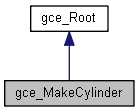# gce_MakeCylinder Class Reference

This class implements the following algorithms used to create a Cylinder from gp. More...

`#include <gce_MakeCylinder.hxx>`

Inheritance diagram for gce_MakeCylinder:[legend]

## Public Member Functions

gce_MakeCylinder (const gp_Ax2 &A2, const Standard_Real Radius)
<A2> is the local cartesian coordinate system of <me>. The status is "NegativeRadius" if R < 0.0 More...

gce_MakeCylinder (const gp_Cylinder &Cyl, const gp_Pnt &Point)
Makes a Cylinder from gp <TheCylinder> coaxial to another Cylinder <Cylinder> and passing through a Pnt <Point>. More...

gce_MakeCylinder (const gp_Cylinder &Cyl, const Standard_Real Dist)
Makes a Cylinder from gp <TheCylinder> coaxial to another Cylinder <Cylinder> at the distance <Dist> which can be greater or lower than zero. The radius of the result is the absolute value of the radius of <Cyl> plus <Dist> More...

gce_MakeCylinder (const gp_Pnt &P1, const gp_Pnt &P2, const gp_Pnt &P3)
Makes a Cylinder from gp <TheCylinder> with 3 points <P1>,<P2>,<P3>. Its axis is <P1P2> and its radius is the distance between <P3> and <P1P2> More...

gce_MakeCylinder (const gp_Ax1 &Axis, const Standard_Real Radius)

gce_MakeCylinder (const gp_Circ &Circ)
Makes a Cylinder by its circular base. Warning If an error occurs (that is, when IsDone returns false), the Status function returns: More...

const gp_CylinderValue () const
Returns the constructed cylinder. Exceptions StdFail_NotDone if no cylinder is constructed. More...

const gp_CylinderOperator () const

operator gp_Cylinder () constPublic Member Functions inherited from gce_Root
Standard_Boolean IsDone () const
Returns true if the construction is successful. More...

gce_ErrorType Status () const
Returns the status of the construction: More...Protected Attributes inherited from gce_Root
gce_ErrorType TheError

## Detailed Description

This class implements the following algorithms used to create a Cylinder from gp.

• Create a Cylinder coaxial to another and passing through a point.
• Create a Cylinder coaxial to another at a distance <Dist>.
• Create a Cylinder with 3 points.
• Create a Cylinder by its axis and radius.
• Create a cylinder by its circular base.

## Constructor & Destructor Documentation

 gce_MakeCylinder::gce_MakeCylinder ( const gp_Ax2 & A2, const Standard_Real Radius )

<A2> is the local cartesian coordinate system of <me>. The status is "NegativeRadius" if R < 0.0

 gce_MakeCylinder::gce_MakeCylinder ( const gp_Cylinder & Cyl, const gp_Pnt & Point )

Makes a Cylinder from gp <TheCylinder> coaxial to another Cylinder <Cylinder> and passing through a Pnt <Point>.

 gce_MakeCylinder::gce_MakeCylinder ( const gp_Cylinder & Cyl, const Standard_Real Dist )

Makes a Cylinder from gp <TheCylinder> coaxial to another Cylinder <Cylinder> at the distance <Dist> which can be greater or lower than zero. The radius of the result is the absolute value of the radius of <Cyl> plus <Dist>

 gce_MakeCylinder::gce_MakeCylinder ( const gp_Pnt & P1, const gp_Pnt & P2, const gp_Pnt & P3 )

Makes a Cylinder from gp <TheCylinder> with 3 points <P1>,<P2>,<P3>. Its axis is <P1P2> and its radius is the distance between <P3> and <P1P2>

 gce_MakeCylinder::gce_MakeCylinder ( const gp_Ax1 & Axis, const Standard_Real Radius )

 gce_MakeCylinder::gce_MakeCylinder ( const gp_Circ & Circ )

Makes a Cylinder by its circular base. Warning If an error occurs (that is, when IsDone returns false), the Status function returns:

• Radius is less than 0.0, or
• Dist is negative and has an absolute value which is greater than the radius of Cyl; or
• gce_ConfusedPoints if points P1 and P2 are coincident.

## Member Function Documentation

 const gp_Cylinder& gce_MakeCylinder::Operator ( ) const
 gce_MakeCylinder::operator gp_Cylinder ( ) const
 const gp_Cylinder& gce_MakeCylinder::Value ( ) const

Returns the constructed cylinder. Exceptions StdFail_NotDone if no cylinder is constructed.

The documentation for this class was generated from the following file: